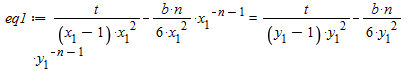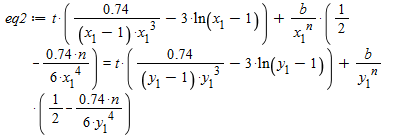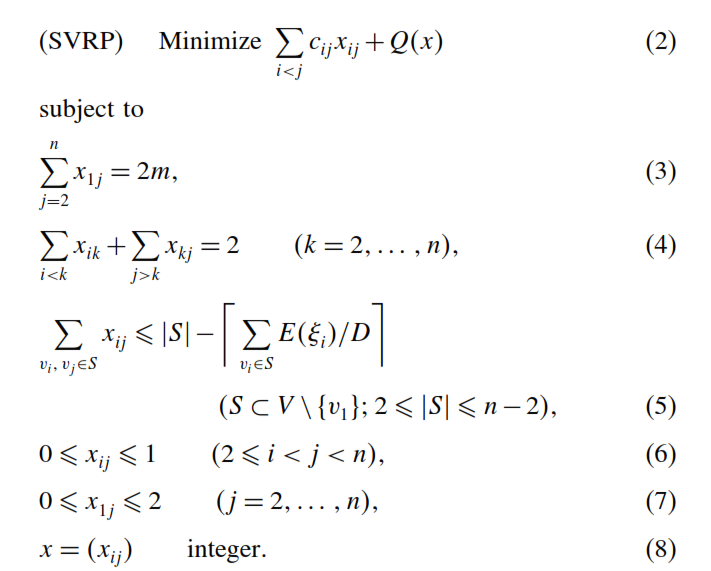## Help: the series is not substituting the previous ...

Good day everyone,

I have a problem building the code attached below. The series is not substituting F(eta) and T(eta).

Please, anyone with useful information should contact.

Thanks

HAM_build.mw

## problem solve a system in maple...i have a problem to solve this system ?

## How can i run the program written by maple 6?...

The program was written in 2004 with maple 6. Now i'am working by maple 18. And i did'nt launch the program. painleve_test.zip

## solve equations and obtain constants...

Hi everybody:
I have 12 equations with 12 unknowns(c(1),c(2),...,c(12) and omega), I want to obtain c(1),c(2),...,c(12) and omega, how can I do it? The Maple file(solve.mw) is attached.
with regards...

solve.mw

## first order non-linear differential equation...

Hello my friends

I want to solve first order non-linear differential equation by maple 2018 but it does not give me the explicit form for a(t) as the function of time t.

this is my equation where z,k,c, and w are non zero constants

3*a(t)-a(t)^2-3*z*(diff(a(t), t))^2+k*c*a(t)+w/a(t) = 0

with the best regard

## Currency convention...

Hi

I would like to designate AU as currency for my application, rather than the USD.

I can do it to a degree, but when I use combine I have problems. Compare output 8 and 9 to 17 and 18. help?

CURRENCY.mw

## Maybe not the right place to tell about that?...

I'm presently interested in PDE and I have just discovered the impressive work Nasser Abbasi,has done, and keeps doing, concerning the solution of PDE benchmarks with Maple and Mathematica.
https://www.12000.org/my_notes/pde_in_CAS/pdse3.htm

It seems that the result Maple returns for the test case below is not the general solution.

• 4.19 first order PDE of three unknowns
problem number 19
(from example 3.5.4, p 212, nonlinear ode’s by Lokenath Debnath, 3rd edition)

It is rather simple to see that any spherical function u(x, y, z) =f(x^2+y^2+z^2) is a solution of the PDE.
Then any function of the form u(x, y, z) = f(x^2+y^2+z^2) * exp(x+y+z) *C1 (C1 being any constant) is also a solution.
Maple returns only the solution u(x, y, z) = exp(C2*(x^2+y^2+z^2)) * exp(x+y+z) * C1

 > restart:
 > u := (x, y, z) -> f(x^2+y^2+z^2)(1)
 > expr := (y-z)*diff(u(x, y, z), x)+(z-x)*diff(u(x, y, z), y)+(x-y)*diff(u(x, y, z), z)(2)
 > simplify(%);(3)
 > u := (x, y, z) -> C*f(x^2+y^2+z^2)*exp(x+y+z)(4)
 > expr := (y-z)*diff(u(x, y, z), x)+(z-x)*diff(u(x, y, z), y)+(x-y)*diff(u(x, y, z), z)(5)
 > simplify(%);(6)
 > pdsolve((y-z)*diff(U(x, y, z), x)+(z-x)*diff(U(x, y, z), y)+(x-y)*diff(U(x, y, z), z)=0, U(x,y,z),'build')(7)
 > combine(rhs(%), exp)(8)
 >

## CVRP Stochastics Demands...

Hello,

I got problem how to rewrite formula in maple, I have tried so many times but it is not working.

How to rewrite sum of double sum like this formula (4th constraints in the following picture)H := numelems(N); for k from 2 to H do for i to k-1 do cons[k] := add(x[i, k], i = N)+add(x[k, j], N) = 2 end do end do;
H := 5
Error, invalid input: add received {1, 2, 3, 4, 5}, which is not valid for its 2nd argument, i

## how to get the integers indicating positions of a ...

for example, there is an expression exp1=(a+b)*(a+c),  I want to find all the position in DAG of a specific operand, such as 'a' ; also I want to find the position in the DAG of  sub-expression which type is '+' .
is there any function in maple which can return the  integers indicating position of an operand or type in an expression? i.e. with this function, assume its name is 'find',then
find(exp1, a) will return  [1,1],[2,1];
find(exp1,'+') will return  [1,0],[2,0];

## 2system of non coupled pde with coupled boundary c...

hi

i got a problem to solve 2 nonlinear  pde equation that are not coupled but there is 8 coupled boundary condition. i can use pdsolve in maple.anyone can help me in numerical solving of these pdes?

## setting range (bounds) to all variables in Minimiz...

Hello

How can I set the range (bounds) of all of the function's variables to the same range at once? Here is a simple exaple:

Input:

Minimize((x-1)^2+(x-1)^2+(x-1)^2, {8 <= x*(x^2+x^2+1)}, x = 1 .. 2, x = 1 .. 2, x = 1 .. 2);

Results:

[0.693290283715211841, [x = 1.53166579937811,  x = 1.45311243719790, x = 1.45311243719790]]

My function have 15 variables x -> x. How can I set their bounds to a global range, say: x[i]=1..2 ?

I'm using Maple 17.3 at work.

Thanks, and sorry for the bad English.

## How to specify multiple lights in plot3d?...

This is from the documentation of ?plot3d,light:

light=[phi, theta, r, g, b]

This option adds [my emphasis] a directed light source

from the direction phi, theta in spherical coordinates.

The "adds" in that sentence seems to imply that

multiple light sources are possible, but it does not seem

to work that way.  Each subsequent light option overrides

the previous one as we see in the following illustration

where we render a ball three times by illuminating it

(a) from the north, (b) from the south, and (c) from

both the north and south.  In (c) Maple accounts for only

the south light.

 > restart;
 > with(plots): with(plottools):
 > ball := sphere(1, style=surface, color=gold):
 > display(<     display(ball,light=[0,0,1,1,1])   |     display(ball,light=[180,0,1,1,1]) |     display(ball,light=[0,0,1,1,1], light=[180,0,1,1,1])  >);## How to use X≔Statistics[RandomVariable](Normal(0,1...

When I try:

X≔Statistics[RandomVariable](Normal(0,1)):
Statistics[Sample](X,10);

I get:

Error, (in Statistics:-Sample) invalid input: expected distribution or algebraic expression with random variables, but received X

What am I doing wrong?

## Parallel tasks writing into multiple files (errors...

Hello,

I am trying to parallelize the output of my Maple18 program, such that it is able to write into multiple smaller output files at the same time, rather than into one unique big file spending much time in this operation...

This is the code:

As you can see from the code, I have tried with different combinations of options. The errors that I get are:

-----------------------------------------

(only one file: "rows-1 < 10000")

Option1A = Error, (in fprintf) string expected for string format

Option1B = Error, (in unspecified) Too many levels of recursion for display

Option2A = OK (but there isn't parallelism here....)

Option2B = OK (but there isn't parallelism here....)

--------

(more than one file: "rows-1 > 10000")

Option1A = Error, (in fprintf) string expected for string format

Option1B = Error, (in unspecified) Too many levels of recursion for display

Option2A = Error, (in StringTools:-Split) first argument must be a string

Option2B = Error, (in StringTools:-Split) first argument must be a string

-----------------------------------------

Instead, if I simplify the definition of D_vol to one single sum (for exemple 2+2), this is what I get:

-----------------------------------------

(only one file: "rows-1 < 10000")

Option1A = OK (but there isn't parallelism here, and the example is trivial 2+2=4)

Option1B = OK (but there isn't parallelism here, and the example is trivial 2+2=4)

Option2A = OK (but there isn't parallelism here, and the example is trivial 2+2=4)

Option2B = OK (but there isn't parallelism here, and the example is trivial 2+2=4)

--------

(more than one file: "rows-1 > 10000")

Option1A = OK (but the example is trivial 2+2=4)

Option1B = OK (but the example is trivial 2+2=4)

Option2A = Error, (in StringTools:-Split) first argument must be a string

Option2B = Error, (in StringTools:-Split) first argument must be a string

-----------------------------------------

Thanks

## print multiple-choice can tab?...